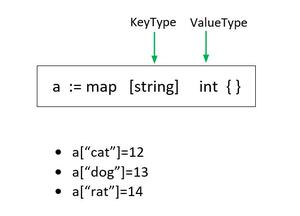Open in App
Not now

# Golang program that removes duplicates, ignores order

• Last Updated : 07 Feb, 2022

In Golang when we want to remove the duplicates not considering any particular order as the initial values, we make use of Mapping in Go lang.Firstly iterate through the loop and map each and every element in the array to boolean data type. Go provides a built-in map type that implements a hash table.

Example: In this example we map string data type to int data type i.e., every string value is associated with integer valueLets understand the program line by line:

```package main
import "fmt"```

Package fmt implements formatted I/O with functions analogous to C’s printf and scanf.

`func unique(arr []int) []int {`

The function definition that we define to remove duplicate elements with the parameter as an input array ‘arr’ and return an array of type ‘[ ]int’

```result:=[]int{}
encountered := map[int]bool{}```

Create an array result that will store all the unique elements.’encountered’ stores the mapped value of integer variables to boolean data type.

```for v:= range arr {
encountered[arr[v]] = true
}```

Iterate through the loop  and for every element that occurs in ‘arr’, we set it to True.The element which are present multiple times have the same hash(or mapped) value.

``` for key, _ := range encountered {
result = append(result, key)
}
return result
}```

Then we iterate through the key and value pairs present in the mapped array.Then we append it to the result array the key elements which stores the unique value.

```func main() {
array1 := []int{1, 5, 3, 4, 1, 6, 6, 6, 8, 7, 13, 5}
fmt.Println(array1)
unique_items := unique(array1)
fmt.Println(unique_items)
}```

Here in the function main we initialize an integer array with some elements having repetition. We print the initial array and the updated array after calling the unique() function. This gives us the unique elements, ignoring any particular order.

## Go

 `package` `main`   `import` `"fmt"`   `func` `unique(arr []int) []int {` `    ``result := []int{}` `    ``encountered := ``map``[int]bool{}`   `    ``// Create a map of all unique elements.` `    ``for` `v := ``range` `arr {` `        ``encountered[arr[v]] = true` `    ``}`   `    ``// Place all unique keys from ` `    ``// the map into the results array.` `    ``for` `key, _ := ``range` `encountered {` `        ``result = append(result, key)` `    ``}` `    ``return` `result` `}`   `func` `main() {` `    ``array1 := []int{``2``, ``4``, ``5``, ``7``, ``2``, ``3``, ``4``,` `             ``7``, ``7``, ``12``, ``5``, ``11``, ``11``, ``1``, ``13``}` `    ``fmt.Println(array1)` `    ``unique_items := unique(array1)` `    ``fmt.Println(unique_items)` `}`

Output:

```[2 4 5 7 2 3 4 7 7 12 5 11 11 1 13]
[11 1 13 2 4 5 7 3 12]```
My Personal Notes arrow_drop_up
Related Articles